## A Few Words on Representation Learning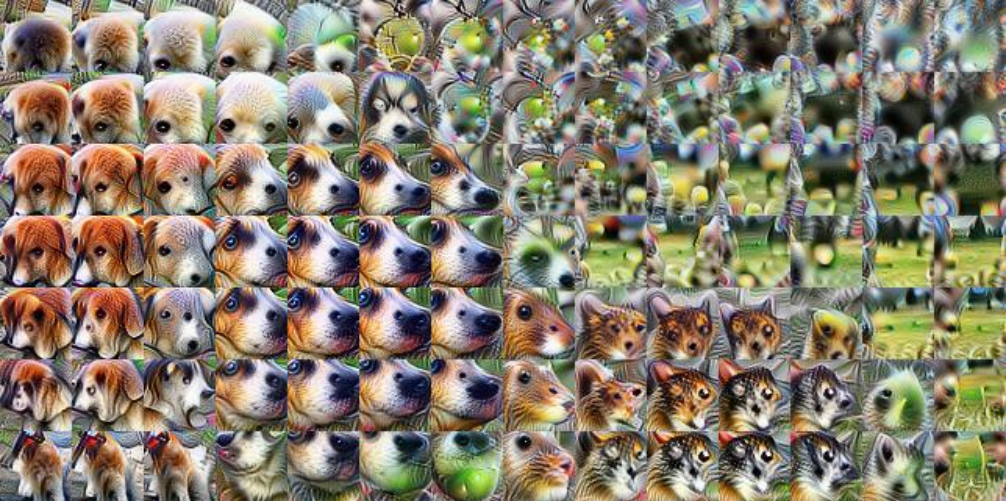Representation learning aims to map high-dimensional data to often dense, compact, and generalizable representations. These learned representations can transfer well to other tasks and have been the principal method to solve problems in which data annotations are hard or even impossible to get.

## Understanding Linear Regression using the Singular Value DecompositionIt is common to see educational material explaining linear regression using gradient descent. In this post, we will take on linear regression through the lens of linear algebra and solve it using the singular value decomposition (SVD).

## Exploring SimCLR: A Simple Framework for Contrastive Learning of Visual Representations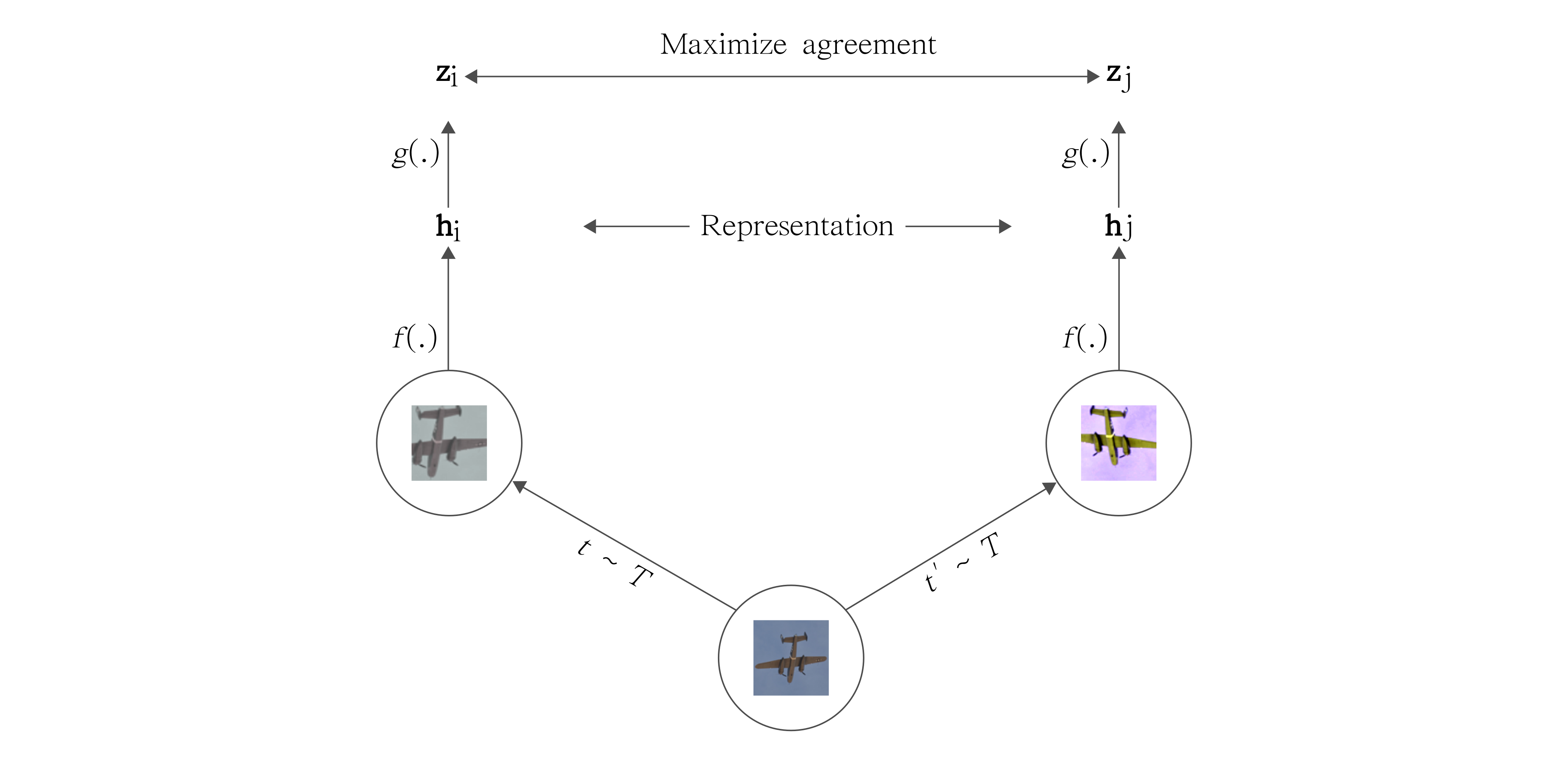A word on contrastive learning and the recent success of unsupervised representation learning. We also build and explore SimCLR: A simple framework for contrastive learning.

## Self-Supervised Learning and the Quest for Reducing Labeled Data in Deep LearningNotes on the current state of deep learning and how self-supervision may be the answer to more robust models

## Practical Deep Learning Audio Denoising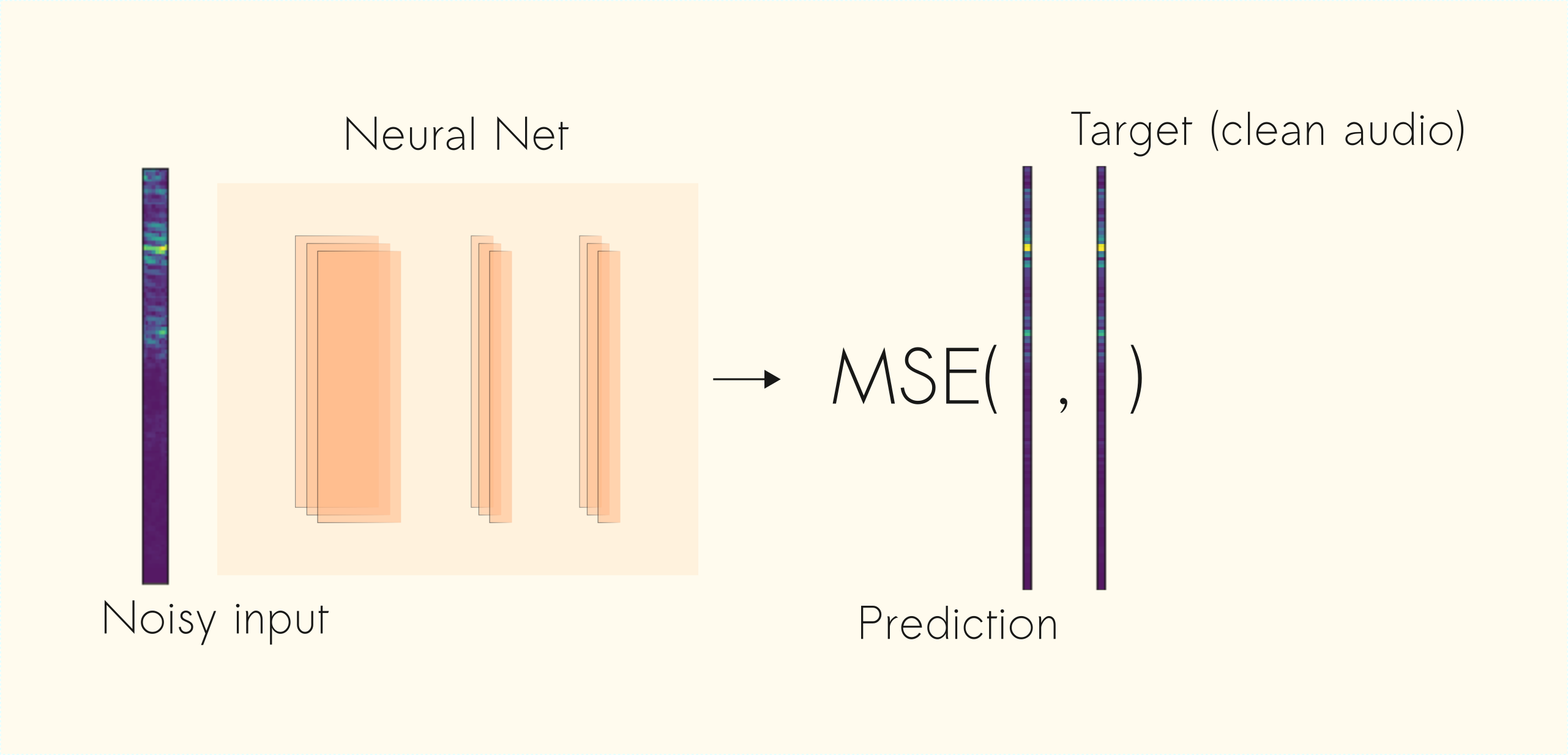Speech denoising is a long-standing problem. In this article, we use Convolutional Neural Networks (CNNs) to tackle this problem. Given a noisy input signal, we aim to build a statistical model that can extract the clean signal (the source) and return it to the user.

## How to Add Regularization to Keras Pre-trained Models the Right WayIf you train deep learning models for a living, you might be tired of knowing one specific and important thing: fine-tuning deep pre-trained models requires a lot of regularization.

## A Visual Guide to Time Series Decomposition Analysis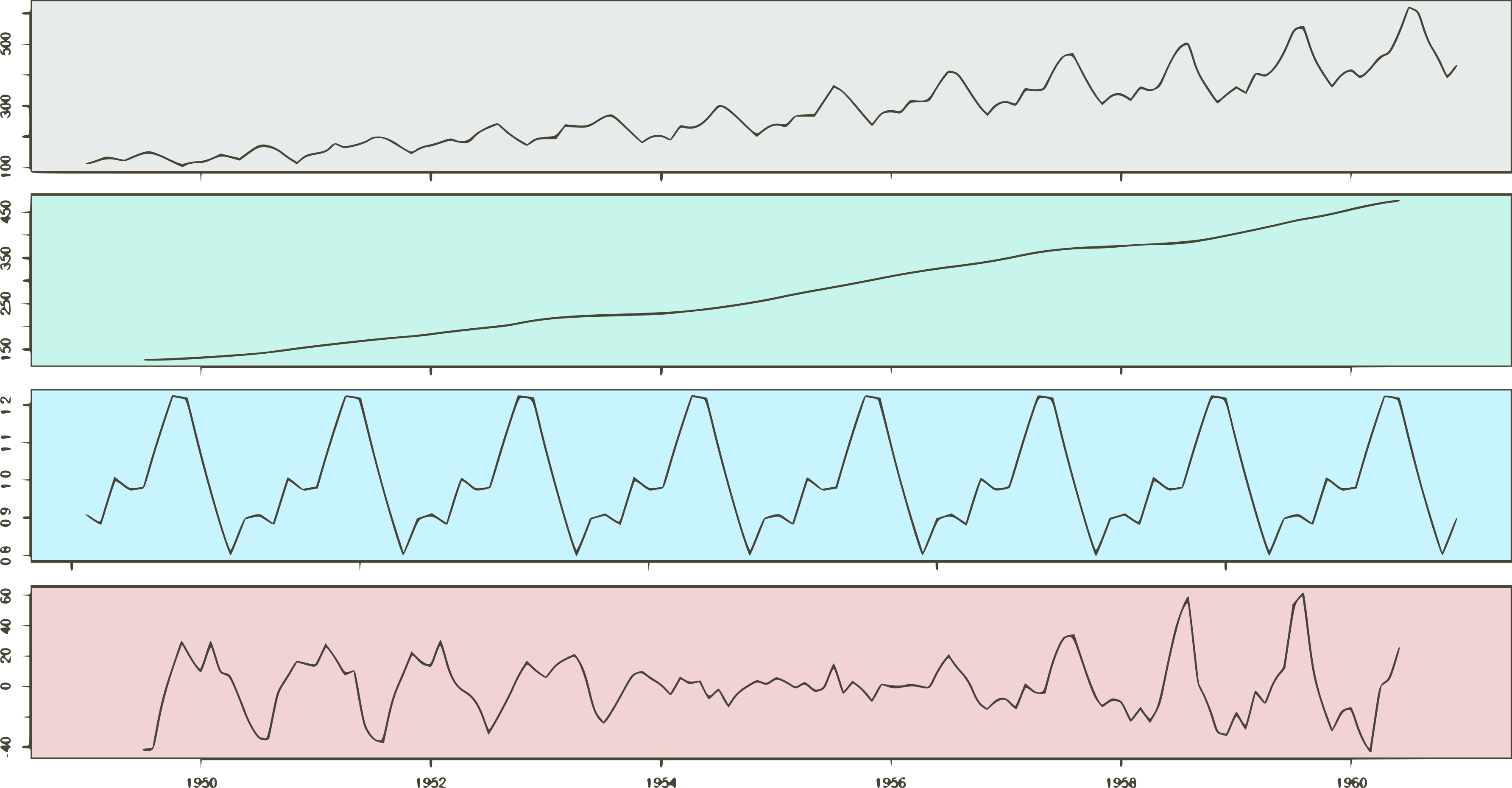Time Series Forecasting is the use of statistical methods to predict future behavior based on a series of past data. Simply put, we can think of it as a bunch of values collected through time. In this post, we explore two decomposition methods: additive and multiplicative decomposition.

## Logistic Regression: The good parts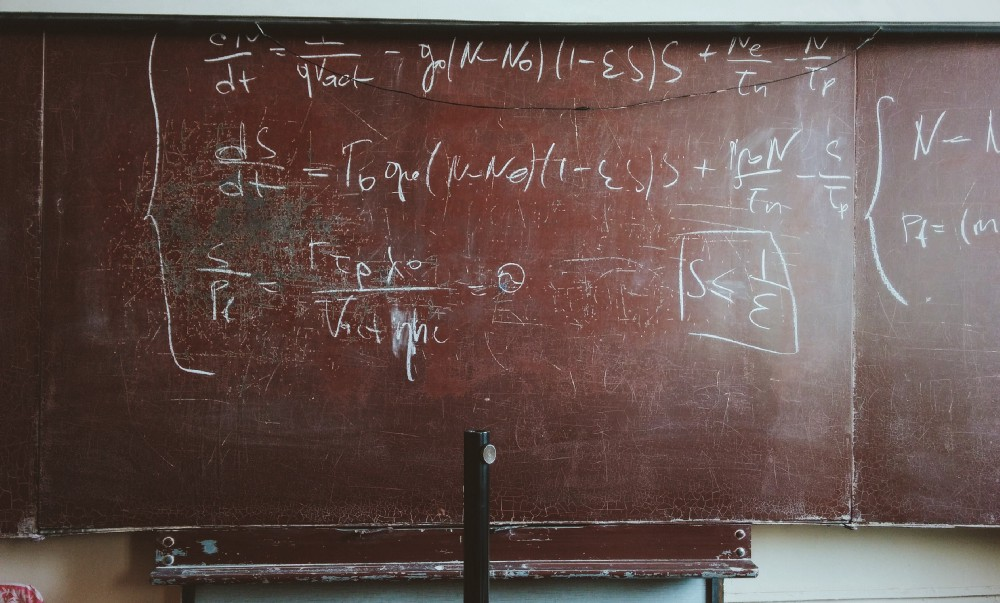We can categorize ML models based on the way they classify data. There are two types: generative and discriminative methods. Let's dive deeper into one of the most popular discriminative models - Logistic Regression.

## An illustrative introduction to Fisher's Linear DiscriminantTo deal with problems with 2 or more classes, most ML algorithms work the same way. Usually, they apply some kind of transformation to the input data. The goal is to project the data to a new space. Then, they try to classify the data points by finding a linear separation.

## Advanced GANs - Exploring Normalization Techniques for GAN training: Self-Attention and Spectral NormLately, Generative Models are drawing a lot of attention. Much of that comes from Generative Adversarial Networks (GANs). Let's investigate some recent techniques for improving GAN training.

## How to deploy TensorFlow models to production using TF ServingPutting Machine Learning (ML) models to production has been a recurrent topic. To address this concern, TensorFlow (TF) Serving is Google’s best bet for deploying ML models to production.

## Deeplab Image Semantic Segmentation NetworkDeep Convolution Neural Networks (DCNNs) have achieved remarkable success in various Computer Vision applications. Like others, the task of semantic segmentation is not an exception to this trend.

## Densely Connected Convolutional Networks in Tensorflow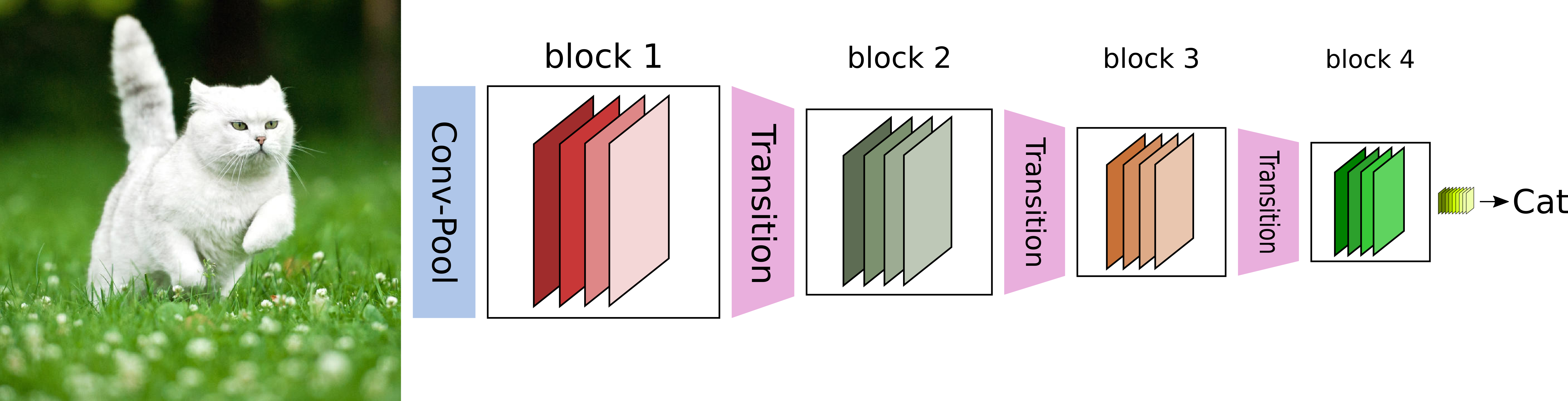DenseNets offer very scalable models that achieve very good accuracy and are easy to train. The key idea consists sharing feature maps within a block through direct connections between layers.

## Semi-supervised Learning with GANsSupervised learning has been the center of most researching in deep learning in recent years. However, the necessity of creating models capable of learning from fewer or no labeled data is greater year by year.

## A Short Introduction to Generative Adversarial NetworksGANs are a kind of generative model in which two differentiable functions are locked in a game. The generator tries to reproduce data that come from some probability distribution. The discriminator gets to decide if its input comes from the generator or from the true training set.